# Analogy Quiz IV, Non Verbal Reasoning

## 20 Questions MCQ Test Non Verbal Reasoning | Analogy Quiz IV, Non Verbal Reasoning

Description
Attempt Analogy Quiz IV, Non Verbal Reasoning | 20 questions in 40 minutes | Mock test for LR preparation | Free important questions MCQ to study Non Verbal Reasoning for LR Exam | Download free PDF with solutions
QUESTION: 1

### Directions to Solve Each of the following questions consists of two sets of figures. Figures A, B, C and D constitute the Problem Set while figures 1, 2, 3, 4 and 5 constitute the Answer Set. There is a definite relationship between figures A and B. Establish a similar relationship between figures C and D by selecting a suitable figure from the Answer Set that would replace the question mark (?) in fig. (D). Question - Select a suitable figure from the Answer Figures that would replace the question mark (?).Problem Figures:                            Answer Figures: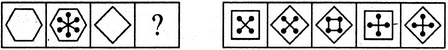(A)     (B)      (C)     (D)                  (1)      (2)      (3)      (4)      (5)

Solution: As many pins are introduced inside the figure as the number of sides of the figure. These pins start from the centre of the figure and have their heads pointing towards the sides of the figure.
QUESTION: 2

### Directions to Solve Each of the following questions consists of two sets of figures. Figures A, B, C and D constitute the Problem Set while figures 1, 2, 3, 4 and 5 constitute the Answer Set. There is a definite relationship between figures A and B. Establish a similar relationship between figures C and D by selecting a suitable figure from the Answer Set that would replace the question mark (?) in fig. (D). Question - Select a suitable figure from the Answer Figures that would replace the question mark (?).Problem Figures:                            Answer Figures: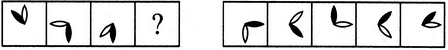(A)     (B)      (C)     (D)                  (1)      (2)      (3)      (4)      (5)

Solution: The black leaf rotates 135oACW and white leaf rotates 90oACW
QUESTION: 3

### Directions to Solve Each of the following questions consists of two sets of figures. Figures A, B, C and D constitute the Problem Set while figures 1, 2, 3, 4 and 5 constitute the Answer Set. There is a definite relationship between figures A and B. Establish a similar relationship between figures C and D by selecting a suitable figure from the Answer Set that would replace the question mark (?) in fig. (D). Question - Select a suitable figure from the Answer Figures that would replace the question mark (?).Problem Figures:                            Answer Figures: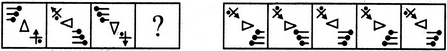(A)     (B)      (C)     (D)                  (1)      (2)      (3)      (4)      (5)

Solution: The set of three pins gets vertically inverted and moves to the lower-right position; the triangle rotates 90oACW; the third element rotates 45oACW and moves to the upper-left position. The dot in this element moves to the adjacent portion in a CW direction.
QUESTION: 4

Each of the following questions consists of two sets of figures. Figures A, B, C and D constitute the Problem Set while figures 1, 2, 3, 4 and 5 constitute the Answer Set. There is a definite relationship between figures A and B. Establish a similar relationship between figures C and D by selecting a suitable figure from the Answer Set that would replace the question mark (?) in fig. (D).

Question -

Select a suitable figure from the Answer Figures that would replace the question mark (?).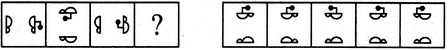(A)     (B)      (C)     (D)                  (1)      (2)      (3)      (4)      (5)
Solution: The RHS element rotates 90oCW and moves to the upper position. The LHS element rotates 90oCW and moves to the lower position.
QUESTION: 5

Each of the following questions consists of two sets of figures. Figures A, B, C and D constitute the Problem Set while figures 1, 2, 3, 4 and 5 constitute the Answer Set. There is a definite relationship between figures A and B. Establish a similar relationship between figures C and D by selecting a suitable figure from the Answer Set that would replace the question mark (?) in fig. (D).

Question -

Select a suitable figure from the Answer Figures that would replace the question mark (?).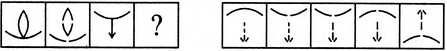(A)     (B)      (C)     (D)                  (1)      (2)      (3)      (4)      (5)
Solution: The arc gets divided at one place and the other element gets separated from the arc and gets divided at two places.
QUESTION: 6

Each of the following questions consists of two sets of figures. Figures A, B, C and D constitute the Problem Set while figures 1, 2, 3, 4 and 5 constitute the Answer Set. There is a definite relationship between figures A and B. Establish a similar relationship between figures C and D by selecting a suitable figure from the Answer Set that would replace the question mark (?) in fig. (D).

Question -

Select a suitable figure from the Answer Figures that would replace the question mark (?).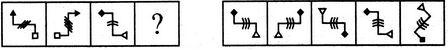(A)     (B)      (C)     (D)                  (1)      (2)      (3)      (4)      (5)
Solution: The figure rotates 90oCW and the number of straight lines or arcs at the centre increases by one.
QUESTION: 7

Each of the following questions consists of two sets of figures. Figures A, B, C and D constitute the Problem Set while figures 1, 2, 3, 4 and 5 constitute the Answer Set. There is a definite relationship between figures A and B. Establish a similar relationship between figures C and D by selecting a suitable figure from the Answer Set that would replace the question mark (?) in fig. (D).

Question -

Select a suitable figure from the Answer Figures that would replace the question mark (?).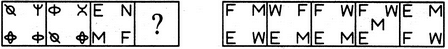(A)     (B)      (C)     (D)                  (1)      (2)      (3)      (4)      (5)
Solution: The symbols move in the sequence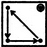and the symbol at the encircled position gets replaced by a new one.
QUESTION: 8

Each of the following questions consists of two sets of figures. Figures A, B, C and D constitute the Problem Set while figures 1, 2, 3, 4 and 5 constitute the Answer Set. There is a definite relationship between figures A and B. Establish a similar relationship between figures C and D by selecting a suitable figure from the Answer Set that would replace the question mark (?) in fig. (D).

Question -

Select a suitable figure from the Answer Figures that would replace the question mark (?).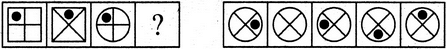(A)     (B)      (C)     (D)                  (1)      (2)      (3)      (4)      (5)
Solution: The contents of the outer main figure rotate through 45oCW.
QUESTION: 9

Each of the following questions consists of two sets of figures. Figures A, B, C and D constitute the Problem Set while figures 1, 2, 3, 4 and 5 constitute the Answer Set. There is a definite relationship between figures A and B. Establish a similar relationship between figures C and D by selecting a suitable figure from the Answer Set that would replace the question mark (?) in fig. (D).

Question -

Select a suitable figure from the Answer Figures that would replace the question mark (?).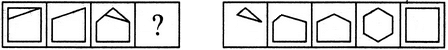(A)     (B)      (C)     (D)                  (1)      (2)      (3)      (4)      (5)
Solution: The upper part of the figure gets separated along the line and is lost.
QUESTION: 10

Each of the following questions consists of two sets of figures. Figures A, B, C and D constitute the Problem Set while figures 1, 2, 3, 4 and 5 constitute the Answer Set. There is a definite relationship between figures A and B. Establish a similar relationship between figures C and D by selecting a suitable figure from the Answer Set that would replace the question mark (?) in fig. (D).

Question -

Select a suitable figure from the Answer Figures that would replace the question mark (?).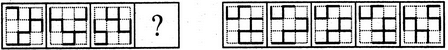(A)     (B)      (C)     (D)                  (1)      (2)      (3)      (4)      (5)
Solution: The figure rotates 90oCW.
QUESTION: 11

Each of the following questions consists of two sets of figures. Figures A, B, C and D constitute the Problem Set while figures 1, 2, 3, 4 and 5 constitute the Answer Set. There is a definite relationship between figures A and B. Establish a similar relationship between figures C and D by selecting a suitable figure from the Answer Set that would replace the question mark (?) in fig. (D).

Question -

Select a suitable figure from the Answer Figures that would replace the question mark (?).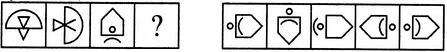(A)     (B)      (C)     (D)                  (1)      (2)      (3)      (4)      (5)
Solution: The figure rotates 90oCW; the inner element rotates about the main line and turns outwards while the outer element shifts inwards,
QUESTION: 12

Each of the following questions consists of two sets of figures. Figures A, B, C and D constitute the Problem Set while figures 1, 2, 3, 4 and 5 constitute the Answer Set. There is a definite relationship between figures A and B. Establish a similar relationship between figures C and D by selecting a suitable figure from the Answer Set that would replace the question mark (?) in fig. (D).

Question -

Select a suitable figure from the Answer Figures that would replace the question mark (?).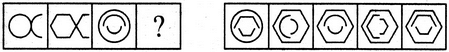(A)     (B)      (C)     (D)                  (1)      (2)      (3)      (4)      (5)
Solution: The circles are converted to hexagons and semicircles are converged to half-hexagons.
QUESTION: 13

Each of the following questions consists of two sets of figures. Figures A, B, C and D constitute the Problem Set while figures 1, 2, 3, 4 and 5 constitute the Answer Set. There is a definite relationship between figures A and B. Establish a similar relationship between figures C and D by selecting a suitable figure from the Answer Set that would replace the question mark (?) in fig. (D).

Question -

Select a suitable figure from the Answer Figures that would replace the question mark (?).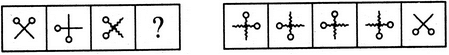(A)     (B)      (C)     (D)                  (1)      (2)      (3)      (4)      (5)
Solution: The figure rotates through 135oACW.
QUESTION: 14

Directions to Solve

Each of the following questions consists of two sets of figures. Figures A, B, C and D constitute the Problem Set while figures 1, 2, 3, 4 and 5 constitute the Answer Set. There is a definite relationship between figures A and B. Establish a similar relationship between figures C and D by selecting a suitable figure from the Answer Set that would replace the question mark (?) in fig. (D).

Question -

Select a suitable figure from the Answer Figures that would replace the question mark (?).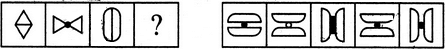(A)     (B)      (C)     (D)                  (1)      (2)      (3)      (4)      (5)

Solution:

The figure rotates through 90o the two parts get separated along the dividing line; each part gets rotated through 180o and these two parts are joined together by a black circle.

QUESTION: 15

Each of the following questions consists of two sets of figures. Figures A, B, C and D constitute the Problem Set while figures 1, 2, 3, 4 and 5 constitute the Answer Set. There is a definite relationship between figures A and B. Establish a similar relationship between figures C and D by selecting a suitable figure from the Answer Set that would replace the question mark (?) in fig. (D).

Question -

Select a suitable figure from the Answer Figures that would replace the question mark (?).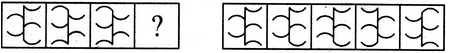(A)     (B)      (C)     (D)                  (1)      (2)      (3)      (4)      (5)
Solution: The figure gets laterally inverted and all the arcs get curved in the opposite direction.
QUESTION: 16

Each of the following questions consists of two sets of figures. Figures A, B, C and D constitute the Problem Set while figures 1, 2, 3, 4 and 5 constitute the Answer Set. There is a definite relationship between figures A and B. Establish a similar relationship between figures C and D by selecting a suitable figure from the Answer Set that would replace the question mark (?) in fig. (D).

Question -

Select a suitable figure from the Answer Figures that would replace the question mark (?).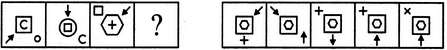(A)     (B)      (C)     (D)                  (1)      (2)      (3)      (4)      (5)
Solution: The arrow rotates 135oCW and moves a distance equal to one and a half sides of the square boundary in a CW direction. Out of the two central elements, the outer element diminishes in size and becomes the inner element, the inner element moves to the position of the corner element and the corner element gets enlarged and moves to the central position to become the outer element.
QUESTION: 17

Each of the following questions consists of two sets of figures. Figures A, B, C and D constitute the Problem Set while figures 1, 2, 3, 4 and 5 constitute the Answer Set. There is a definite relationship between figures A and B. Establish a similar relationship between figures C and D by selecting a suitable figure from the Answer Set that would replace the question mark (?) in fig. (D).

Question -

Select a suitable figure from the Answer Figures that would replace the question mark (?).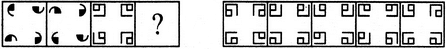(A)     (B)      (C)     (D)                  (1)      (2)      (3)      (4)      (5)
Solution: The upper-left and the lower-right elements rotate 90oCW while the upper-right and the lower-left elements rotate 90oACW.
QUESTION: 18

Each of the following questions consists of two sets of figures. Figures A, B, C and D constitute the Problem Set while figures 1, 2, 3, 4 and 5 constitute the Answer Set. There is a definite relationship between figures A and B. Establish a similar relationship between figures C and D by selecting a suitable figure from the Answer Set that would replace the question mark (?) in fig. (D).

Question -

Select a suitable figure from the Answer Figures that would replace the question mark (?).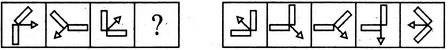(A)     (B)      (C)     (D)                  (1)      (2)      (3)      (4)      (5)
Solution: The arrow rotates 135oCW and the remaining part of the figure rotates 90oACW.
QUESTION: 19

Each of the following questions consists of two sets of figures. Figures A, B, C and D constitute the Problem Set while figures 1, 2, 3, 4 and 5 constitute the Answer Set. There is a definite relationship between figures A and B. Establish a similar relationship between figures C and D by selecting a suitable figure from the Answer Set that would replace the question mark (?) in fig. (D).

Question -

Select a suitable figure from the Answer Figures that would replace the question mark (?).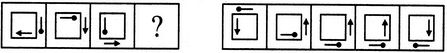(A)     (B)      (C)     (D)                  (1)      (2)      (3)      (4)      (5)
Solution: The outer element rotates 90oACW; moves to the adjacent side of the square in an ACW direction and shifts inside the square. The inner element rotates 90oACW; moves to the adjacent side of the square in an ACW direction and shifts outside the square.
QUESTION: 20

Each of the following questions consists of two sets of figures. Figures A, B, C and D constitute the Problem Set while figures 1, 2, 3, 4 and 5 constitute the Answer Set. There is a definite relationship between figures A and B. Establish a similar relationship between figures C and D by selecting a suitable figure from the Answer Set that would replace the question mark (?) in fig. (D).

Question -

Select a suitable figure from the Answer Figures that would replace the question mark (?).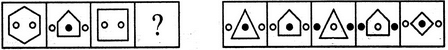Use Code STAYHOME200 and get INR 200 additional OFF Use Coupon Code